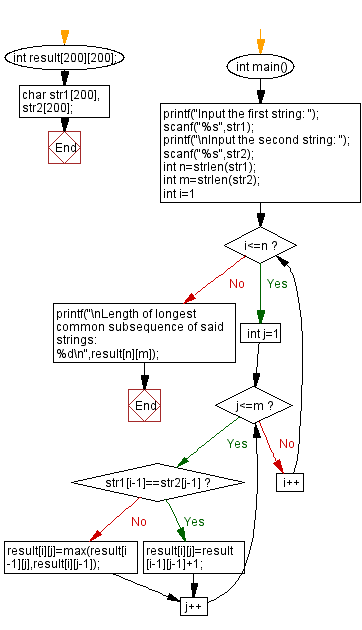﻿ C - Length of longest common subsequence of two strings# C Programming: Length of longest common subsequence of two strings

## C String: Exercise-41 with Solution

Write a C programming to calculate the length of longest common subsequence of two given strings. The strings consist of alphabetical characters.

Sample Data:

("abcdkiou", "cabsdf") -> 3
("pqrjad", "qr") -> 2

Sample Solution:

C Code:

``````#include <stdio.h>
#include <string.h>

#define max(x,y)((x)>(y)?(x):(y))
int result;
char str1, str2;
int main(){
printf("Input the first string: ");
scanf("%s",str1);
printf("\nInput the second string: ");
scanf("%s",str2);
int n=strlen(str1);
int m=strlen(str2);
for(int i=1;i<=n;i++)for(int j=1;j<=m;j++)
{
if(str1[i-1]==str2[j-1])
result[i][j]=result[i-1][j-1]+1;
else
result[i][j]=max(result[i-1][j],result[i][j-1]);
}
printf("\nLength of longest common subsequence of said strings: %d\n",result[n][m]);
}
```
```

Sample Output:

```Input the first string:
Input the second string:
Length of longest common subsequence of said strings: 3
```

Flowchart :C Programming Code Editor:

Improve this sample solution and post your code through Disqus.

Previous C Programming Exercise: Replace every lowercase letter with the same uppercase.
Next C Programming Exercise: C Date Time Exercises Home

What is the difficulty level of this exercise?

Test your Programming skills with w3resource's quiz.

﻿